• ## 卷积和傅里叶变换

千次阅读 2017-11-29 17:20:49
我们最常接触到的『信号』是一维时间信号，比如人的声音、乐曲、无线电波等，横坐标是...把信号从『时域』映射到『频域』的手段，就是大家耳熟能详的『傅里叶变换』。  虽然我们一直在强调『时间信号』，但不知大家
我们最常接触到的『信号』是一维时间信号，比如人的声音、乐曲、无线电波等，横坐标是时间t，纵坐标是信号的幅度，代表不同时刻的信号强度。

如果我们把一个信号各个频段的成分也画出来，横坐标是频段的『大小』，纵坐标是对应频段成分的『幅度』，这样一个坐标系，我们把它叫做『频域』。把信号从『时域』映射到『频域』的手段，就是大家耳熟能详的『傅里叶变换』。
虽然我们一直在强调『时间信号』，但不知大家注意到没有，其实这里的时间t，完全可以换成其他符号比如x，从而所谓的时间信号f(t)，可以写成f(x)，进而，图像可以看成一个离散的二维函数f(x,y)，x 和 y 决定了图像的像素点，f是像素点在该处的取值。更形象地理解，图像就仿佛是一个『水池』，像素点就是『水分子』，像素点的取值大小，从视觉上看代表图像亮度的强弱，而类比到水池里，就是不同位置水分子的运动幅度，在水池里泛起涟漪。
进而，我们很自然地想到，一维函数的『傅里叶变换』，能否扩展到二维呢？
答案是肯定的。不过二维空间的傅里叶变换公式我们就不贴出来了，大家有兴趣可以详细阅读参考资料。一维函数f(x)的频谱函数F(w)，是一维信号的不同频率分量，而二维函数f(x,y)的频谱函数，是一个二维函数F(w,v)，也反应了二维函数的频率特性(不过理解起来不那么直观，这里略过
展开全文• 卷积傅里叶变换攻略-卷积傅里叶变换攻略.pdf 很犀利的一片文章，阐述的很清楚！matlab
• 图像的卷积傅里叶变换、小波变换基础。PPT，很基础，很清晰。
• ## 卷积与傅里叶变换

千次阅读 2018-11-07 11:30:16
卷积傅里叶变换
分享一下我老师大神的人工智能教程！零基础，通俗易懂！http://blog.csdn.net/jiangjunshow

也欢迎大家转载本篇文章。分享知识，造福人民，实现我们中华民族伟大复兴！

很多朋友和我一样，工科电子类专业，学了一大堆信号方面的课，什么都没学懂，背了公式考了试，然后毕业了。
先说"卷积有什么用"这个问题。(有人抢答，"卷积"是为了学习"信号与系统"这门课的后续章节而存在的。我大吼一声，把他拖出去枪毙！)
讲一个故事:
张三刚刚应聘到了一个电子产品公司做测试人员，他没有学过"信号与系统"这门课程。一天，他拿到了一个产品，开发人员告诉他，产品有一个输入端，有一个输出端，有限的输入信号只会产生有限的输出。
然后，经理让张三测试当输入sin(t)(t<1秒)信号的时候(有信号发生器)，该产品输出什么样的波形。张三照做了，画了一个波形图。
"很好！"经理说。然后经理给了张三一叠A4纸: "这里有几千种信号，都用公式说明了，输入信号的持续时间也是确定的。你分别测试以下我们产品的输出波形是什么吧！"
这下张三懵了，他在心理想"上帝，帮帮我把，我怎么画出这些波形图呢?"
于是上帝出现了: "张三，你只要做一次测试，就能用数学的方法，画出所有输入波形对应的输出波形"。
上帝接着说:"给产品一个脉冲信号，能量是1焦耳，输出的波形图画出来！"
张三照办了，"然后呢?"
上帝又说，"对于某个输入波形，你想象把它微分成无数个小的脉冲，输入给产品，叠加出来的结果就是你的输出波形。你可以想象这些小脉冲排着队进入你的产品，每个产生一个小的输出，你画出时序图的时候，输入信号的波形好像是反过来进入系统的。"
张三领悟了:" 哦，输出的结果就积分出来啦！感谢上帝。这个方法叫什么名字呢?"
上帝说:"叫卷积！"
从此，张三的工作轻松多了。每次经理让他测试一些信号的输出结果，张三都只需要在A4纸上做微积分就是提交任务了！
----------------------------------------
张三愉快地工作着，直到有一天，平静的生活被打破。
经理拿来了一个小的电子设备，接到示波器上面，对张三说: "看，这个小设备产生的波形根本没法用一个简单的函数来说明，而且，它连续不断的发出信号！不过幸好，这个连续信号是每隔一段时间就重复一次的。张三，你来测试以下，连到我们的设备上，会产生什么输出波形！"
张三摆摆手:"输入信号是无限时长的，难道我要测试无限长的时间才能得到一个稳定的，重复的波形输出吗?"
经理怒了:"反正你给我搞定，否则炒鱿鱼！"
张三心想:"这次输入信号连公式都给出来，一个很混乱的波形；时间又是无限长的，卷积也不行了，怎么办呢?"
及时地，上帝又出现了:"把混乱的时间域信号映射到另外一个数学域上面，计算完成以后再映射回来"
"宇宙的每一个原子都在旋转和震荡，你可以把时间信号看成若干个震荡叠加的效果，也就是若干个可以确定的，有固定频率特性的东西。"
"我给你一个数学函数f，时间域无限的输入信号在f域有限的。时间域波形混乱的输入信号在f域是整齐的容易看清楚的。这样你就可以计算了"
"同时，时间域的卷积在f域是简单的相乘关系，我可以证明给你看看"
"计算完有限的程序以后，取f(-1)反变换回时间域，你就得到了一个输出波形，剩下的就是你的数学计算了！"
张三谢过了上帝，保住了他的工作。后来他知道了，f域的变换有一个名字，叫做傅里叶，什么什么... ...
----------------------------------------
再后来，公司开发了一种新的电子产品，输出信号是无限时间长度的。这次，张三开始学拉普拉斯了......
PS：
不是我们学的不好，是因为教材不好，老师讲的也不好。

给我老师的人工智能教程打call！http://blog.csdn.net/jiangjunshow展开全文• 卷积在一个线性移不变系统中, 卷积运算可以看做是该系统对输入信号 f(x,y)f(x,y) 的响应.用符号 ⊗\otimes 表示卷积, 则有: g=f⊗h g = f \otimes h 系统的输出响应 gg 是输入信号 ff 与系统函数 hh 做卷积运算...
卷积
在一个线性移不变系统中, 卷积运算可以看做是该系统对输入信号

f(x,y)
$f(x,y)$ 的响应.用符号

⊗
$\otimes$ 表示卷积, 则有:

g=f⊗h

g = f \otimes h

系统的输出响应

g
$g$ 是输入信号 f$f$ 与系统函数

h
$h$ 做卷积运算的结果. 那该如何做呢? 我们知道函数 f(x,y)$f(x,y)$ 可以分解为无限的冲击函数乘以对应权值

f(ξ,η)
$f(\xi,\eta)$的加和, 对应的积分形式为:

f(x,y)=∫∞−∞∫∞−∞f(ξ,η)δ(x−ξ,y−η)dξdη(1)

f(x,y) = \int_{-\infty}^{\infty}\int_{-\infty}^{\infty}f(\xi,\eta)\delta(x-\xi,y-\eta)\mathrm{d}\xi\mathrm{d}\eta \tag{1}

而

f(ξ,η)δ(x−ξ,y−η)
$f(\xi,\eta)\delta(x-\xi,y-\eta)$ 可以看做是经过了
位移和
尺度变换的冲击函数. 那为什么要用到冲击函数呢? 这是因为冲击函数有个很好的性质, 它与系统函数

h
$h$ 做卷积得到的还是系统函数本身:
∫∞−∞∫∞−∞δ(x−ξ,y−η)h(ξ,η)dξdη=h(x,y)
\int_{-\infty}^{\infty}\int_{-\infty}^{\infty}\delta(x-\xi,y-\eta)h(\xi,\eta)\mathrm{d}\xi\mathrm{d}\eta = h(x,y)

即

δ(x,y)
$\delta(x, y)$ 的响应是

h(x,y)
$h(x,y)$ ,对于线性移不变系统,满足:

f(ξ,η)δ(x−ξ,y−η)
$f(\xi,\eta)\delta(x-\xi,y-\eta)$ 的响应是

f(ξ,η)h(x−ξ,y−η)
$f(\xi,\eta)h(x-\xi,y-\eta)$ 我们由式

(1)
$(1)$知道对

f(ξ,η)δ(x−ξ,y−η)
$f(\xi,\eta)\delta(x-\xi,y-\eta)$ 求积是输入信号

f(x,y)
$f(x,y)$ ,同理, 我们对

f(ξ,η)h(x−ξ,y−η)
$f(\xi,\eta)h(x-\xi,y-\eta)$ 求积便得到了响应

g(x,y)
$g(x,y)$:

g(x,y)=∫∞−∞∫∞−∞f(ξ,η)h(x−ξ,y−η)dξdη(2)

g(x,y) = \int_{-\infty}^{\infty}\int_{-\infty}^{\infty}f(\xi,\eta)h(x-\xi,y-\eta)\mathrm{d}\xi\mathrm{d}\eta \tag{2}

注意式

(2)
$(2)$ 对应的是

g=h⊗f
$g = h \otimes f$ 不过我们易证得卷积运算是满足交换律的.

傅里叶变换
还是从信号分解说起, 我们知道输入信号

f(x,y)
$f(x,y)$ 可以由无限冲击函数的加权和来表示, 其实它还有一种分解的方法:

f(x,y)=14π2∫∞−∞∫∞−∞F(u,v)e+i(ux+vy)dudv(3)

f(x,y) = \frac{1}{4\pi^2}\int_{-\infty}^{\infty}\int_{-\infty}^{\infty}F(u,v)e^{+i(ux+vy)}\mathrm{d}u\mathrm{d}v \tag{3}

那么这种方法能否让我们更容易的求解系统响应呢?我们首先来看看当输入是

ei(ux+vy)
$e^{i(ux+vy)}$ 时, 系统的响应:

g(x,y)=∫∞−∞∫∞−∞e+i[u(x−ξ)+v(y−η)] h(ξ,η)dξdη=ei(ux+vy)∫∞−∞∫∞−∞e−i(uξ+vη)h(ξ,η)dξdηH(u,v)

\begin{align}
g(x,y) &= \int_{-\infty}^{\infty}\int_{-\infty}^{\infty}e^{+i[u(x-\xi)+v(y-\eta)]}\ h(\xi,\eta)\mathrm{d}\xi\mathrm{d}\eta \\
&= e^{i(ux+vy)}\underbrace{\int_{-\infty}^{\infty}\int_{-\infty}^{\infty}e^{-i(u\xi+v\eta)}h(\xi,\eta)\mathrm{d}\xi\mathrm{d}\eta}_{H(u,v)}
\end{align}

从上面的式子可以看出, 若输入为

f(x,y)=ei(ux+vy)
$f(x,y) = e^{i(ux+vy)}$ , 则系统的响应为

g(x,y)=H(u,v)f(x,y)
$g(x,y) = H(u,v)f(x,y)$ 而从式

(3)
$(3)$ 可以看出, 对于任意输入信号都可以表示为

ei(ux+vy)
$e^{i(ux+vy)}$ 加权和的形式.因此由系统的线性移不变性质, 我们可以得到系统的响应为:

g(x,y)=14π2∫∞−∞∫∞−∞H(u,v)F(u,v)e+i(ux+vy)dudv

g(x,y) = \frac{1}{4\pi^2}\int_{-\infty}^{\infty}\int_{-\infty}^{\infty}H(u,v)F(u,v)e^{+i(ux+vy)}\mathrm{d}u\mathrm{d}v

这正好对应了将系统响应从空间域

g(x,y)
$g(x,y)$ 变为频率域

G(x,y)
$G(x,y)$ ,即

G(x,y)=H(u,v)F(u,v)

G(x,y) = H(u,v)F(u,v)

小结
我们从上面的分析中可以看出, 系统响应在空间域中可以看做是输入信号与系统函数做卷积得到,即:

g(x,y)=f(x,y)⊗h(x,y)

g(x,y) = f(x,y) \otimes h(x,y)

而在频率域则可以看做是输入信号与系统函数做乘积得到, 即:

G(x,y)=H(u,v)F(u,v)

G(x,y) = H(u,v)F(u,v)

把信号从空间域变到频率域称为
傅里叶变换:

F(u,v)=∫∞−∞∫∞−∞f(x,y)e−i(ux+vy)dudv

F(u,v) = \int_{-\infty}^{\infty}\int_{-\infty}^{\infty}f(x,y)e^{-i(ux+vy)}\mathrm{d}u\mathrm{d}v

对应的, 把信号从频率域变到空间域称为
傅里叶逆变换:

f(x,y)=14π2∫∞−∞∫∞−∞F(u,v)e+i(ux+vy)dudv

f(x,y) = \frac{1}{4\pi^2}\int_{-\infty}^{\infty}\int_{-\infty}^{\infty}F(u,v)e^{+i(ux+vy)}\mathrm{d}u\mathrm{d}v


展开全文• 傅里叶变换的性质 傅里叶对 总结 傅里叶变换的性质 让我们继续看看空间域频域之间的关系。这是傅里叶变换的一些简单性质。我们从一个我们已经讨论过的问题开始。一切都是线性的因为我们只是做求和乘法（如图...
目录
傅里叶变换的性质
傅里叶对
总结

傅里叶变换的性质
让我们继续看看空间域和频域之间的关系。这是傅里叶变换的一些简单性质。我们从一个我们已经讨论过的问题开始。一切都是线性的因为我们只是做求和和乘法（如图），对吧?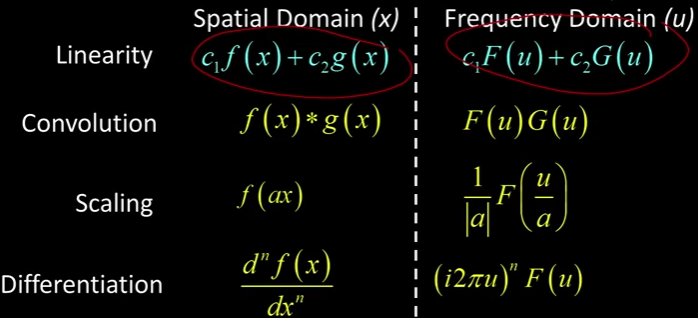我们刚才描述的是这个卷积。空间域的卷积就是频域的乘法，反之亦然，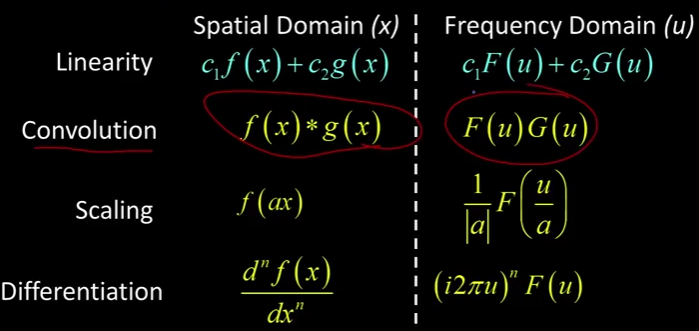一个有趣事情的是缩放。所以，如果我用一个常数a来缩放一个函数。我们这样想，假设a大于1，假设a是2， 这意味着当x = 1时，x = 2，它会收缩，对吧? 当你乘以一个大于1的数，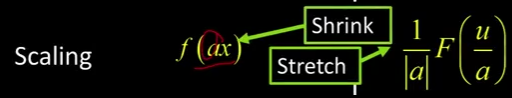如果我有一个函数（如图1），现在发生的是这个值被移到这里（如图2），所以我实际上缩小了这个函数（图3），对吧?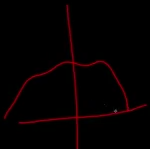1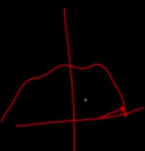2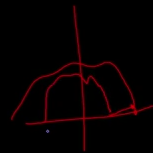3
所以当我缩小一个函数的时候它会摆动得更快，对吧?因为他们之前摆动得比较慢。如果我把它拉进去，它现在摆动得更快。结果是，我拉伸了傅里叶变换（如图）。这就是它的公式，1 / a乘以F (u / a)它有逆性质。就像高斯函数一样，对吧? 如果我让它变得更胖，我的傅立叶变换会变得更瘦。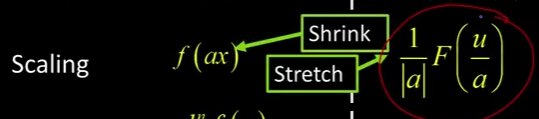最后一个看起来，我们不会使用这么多，但我希望你能看到这个（如图）。当你对一个函数求导时，这是一个n阶导数。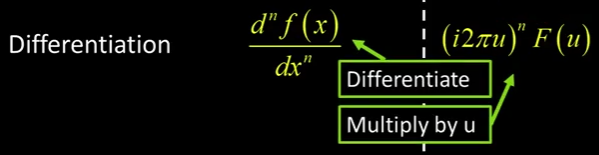你将傅里叶变换F（u）乘以一个与频率成正比的函数（i2πu）^n，所以假设n = 1然后求一阶导数，对吧?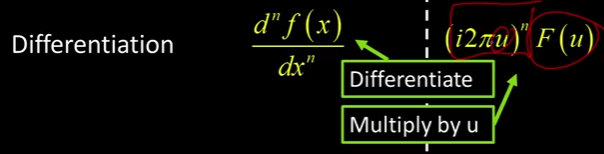也就是说，你要把F (u)乘以u，这就意味着频率越高越明显。这就是求导时的结果。还记得我们加了噪声，高频噪声，然后求导，整个东西都爆炸了吗?那是因为你把它乘以，当你求导时，你乘以高频分量。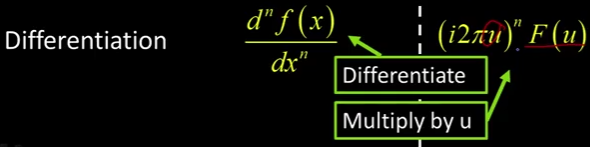傅里叶对
如果你看一下我们前面提到过的Rick Szeliski的书，你可以看到一大堆傅里叶变换对。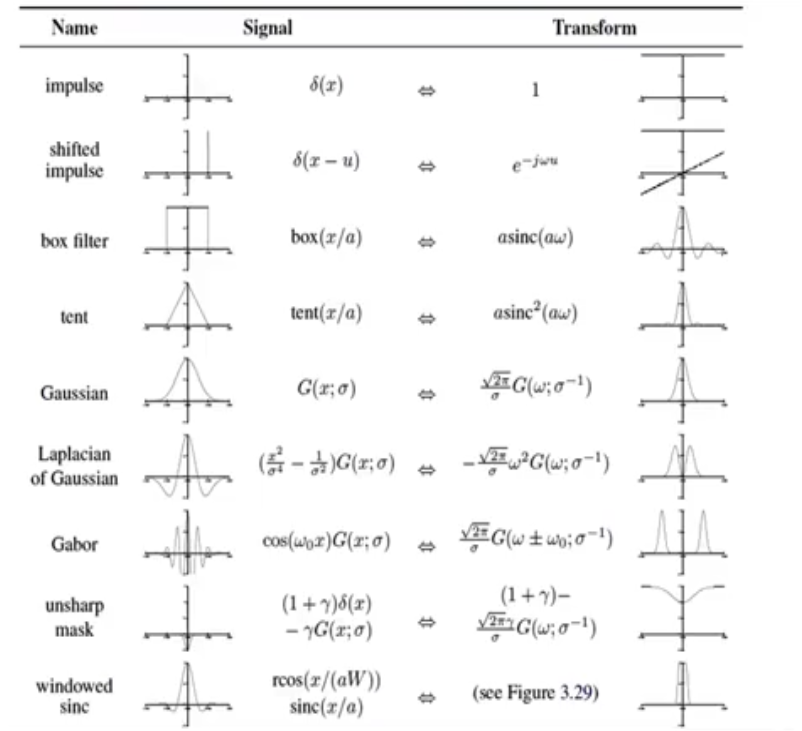例如，像这样绘制的脉冲具有1的傅里叶变换（如图）。就像我们说的那样，这是有道理的，因为当你对它进行卷积时，脉冲会返回原来的函数，所以你最好保持所有的频率。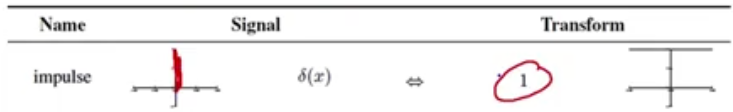同样地，我们说过高斯函数的傅里叶变换是另一个高斯函数（如图）。G()等于1 /。空间上是胖高斯，频率上是瘦高斯。但是看看这个。但是看看这个。如果我有一个盒子过滤器，它只是一个形状像盒子的过滤器，它会产生所谓的sinc函数。画在这里,我们再仔细看看，Okay? 在这个空间域中（左图1），我们有一个方框滤波器这是sinc函数（右图2）。然后sinc(x) = sin(x)/x，我们可以这样想，如果你取x趋于0时的极限，你记得洛必达法则对顶部求导就是余弦，底部的导数就是0中的1，它是1，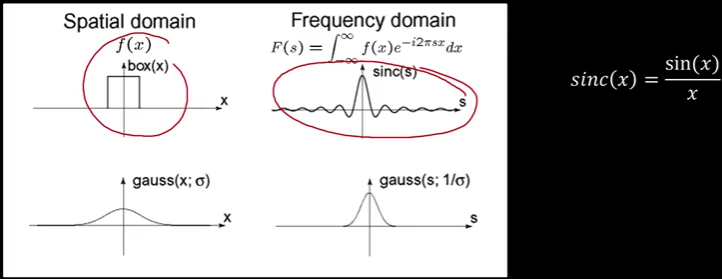x趋于0时的极限是1，在这里，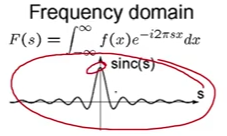然后随着x变大，sin变弱这就是你在这里看到的。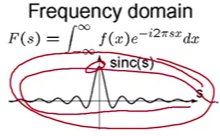换句话说，如果我要用盒子滤波器卷积某些东西。我的频率会以某种奇怪的方式表现出来。你知道，我有这种很好的低通特性（如图），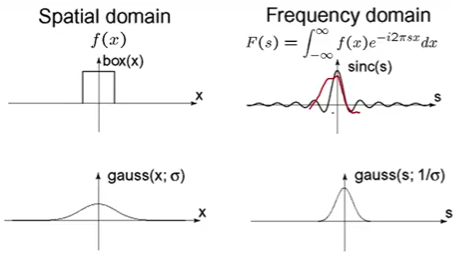但是接下来，我会有各种各样的其他频率（如图），这些频率会加重。如果你还记得，我们在引入过滤时很早就做到了这一点。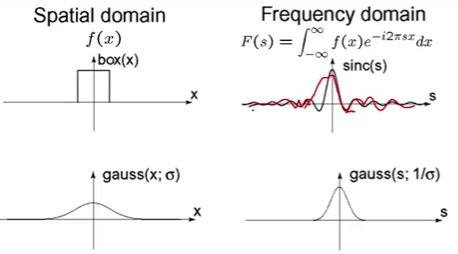还记得吗，我们用一个盒子，和一个正方形做卷积? 我们说，天哪，这么丑吗?这就是为什么它很丑。看到这张图了吗?（如图） 你看它是怎么有这些小条纹的。我说过，当我们第一次做这个的时候，那是因为盒子不是光滑的当我们做傅里叶分析的时候我们会使它更正式。我遵守了我的诺言。 Okay?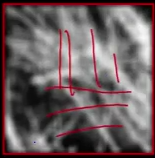当你把一个图像和那个小方块卷积的时候你会看到所有这些垃圾是因为频域中的这个振铃效应（如图）。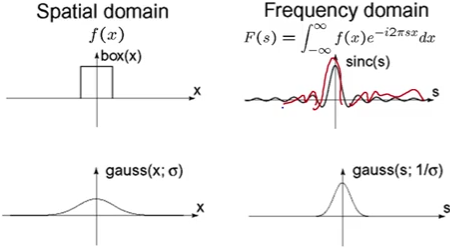这就是为什么我们说，你应该做什么? 你应该应用一个高斯函数而不是一个盒子，它会形成一个漂亮的图像，你知道，一个漂亮的，平滑的图像。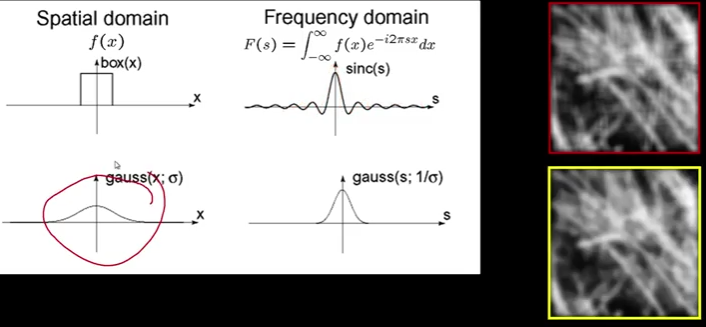因为在频域中，你会得到一个平滑的高斯分布（如图），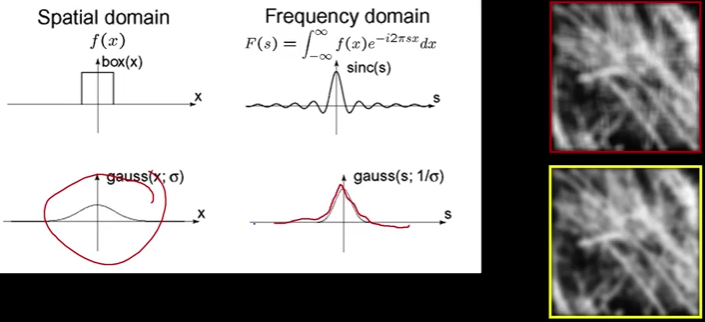关于这些sinc函数还有一件事，好吗？ 所以我们证明了盒子的傅立叶变换（左图1）是sinc（右图1）。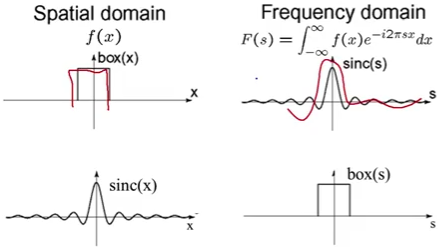好吧，由于对称性，如果我在频率空间中有一个盒子（右图2），那将是空间域中的一个sinc（左图2）。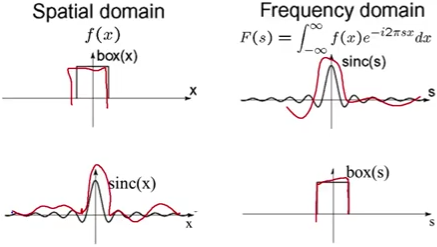那么，如果我要采取一些函数，采用其傅立叶频谱并将其乘以这个方框，那会是什么样的呢？ 好吧，我已经给你们看过了，对吧? 基本上，它有时被称为药盒，因为它是一个盒子。，但在2D中，这不是一个特定的宽度（左图），而是一个半径（右图），对吧？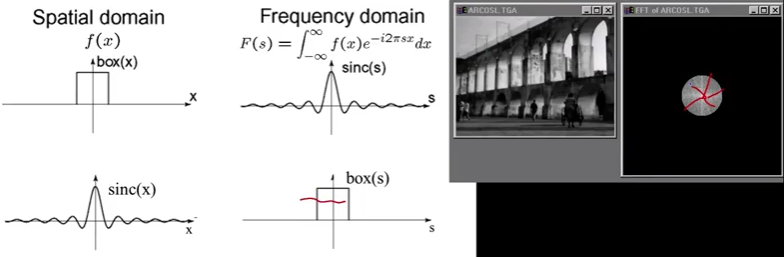因此，直径以外的所有内容都设置为0，内部的所有内容都保持不变。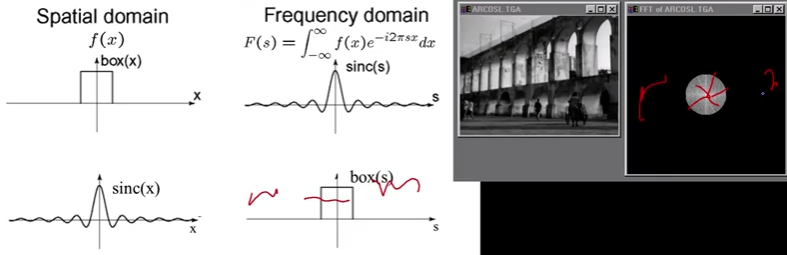如果你还记得我们得到的图片是这张丑陋的图片里面有很多铃声。 好吧，现在我们知道为什么了。 当您通过一个盒子乘以频率时，就好像您使用sinc函数将图像与其傅里叶对进行卷积。 因此，如果你拍摄这张漂亮的照片并用这个丑陋的野兽卷积，你会得到一些看起来像这样的东西（如图）。 这就是振铃的来源。 这就是为什么你永远不想只是削减高频，因为你实际上正在做的是用sinc函数卷积你的图像。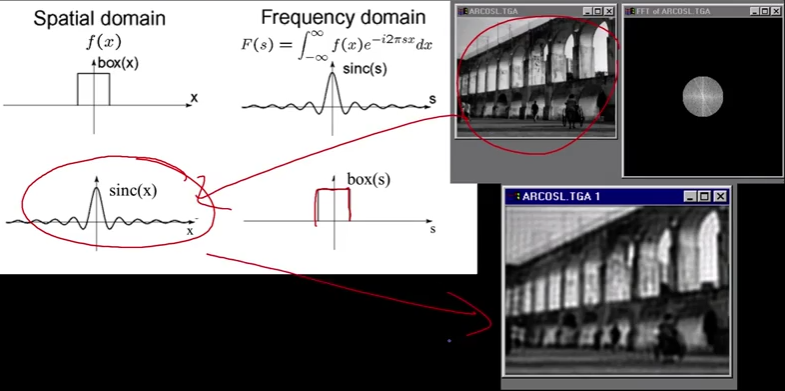加深理解文章：
https://betterexplained.com/articles/an-interactive-guide-to-the-fourier-transform/
总结
这节结束了空间域卷积和频域卷积的关系。特别是空间域的卷积就是频域的乘法。我们也很好地解释了一些丑陋的人为现象，我们看到特殊的振铃是一种盒子的傅里叶变换，如果你很难切断频率，你会得到这个振铃，或者如果你很难在空间上有一个隔断，你会得到这个频率的振铃。我们现在拥有的工具将允许我们处理混叠。当你对一幅图像进行采样时，它会变得非常难看。还有高频的东西。这是一个有点颠簸的旅程，所以带上你自己，准备好下一个内容。

——学会编写自己的代码，才能练出真功夫。
展开全文机器视觉
• 很多朋友我一样，工科电子类专业，学了一大堆信号方面的课，什么都没学懂，背了公式考了试，然后毕业了。  先说"卷积有什么用"这个问题。(有人抢答，"卷积"是为了学习"信号与系统"...
• 解释：空间域中两个函数的卷积傅里叶变换等于两个函数的傅里叶变换在频率域中的乘积；反过来，如果有两个变换的乘积，那么我们可以通过计算傅里叶变换得到空间域的卷积； 是傅里叶变换对； 卷积定理的另一半...
• 转于某博客 很多朋友我一样，工科电子类专业，学了一大堆信号方面的课，什么都没学懂，背了公式考了试，然后毕业了。  先说"卷积有什么用"这个问题。(有人抢答，"卷积"是为了学习"信号与系统"这...测试 数学计算 google
• C++ opencv 卷积 傅里叶变换 程序 废话不多说，直接上代码。 因为两个函数卷积傅里叶变换等于两个函数傅里叶变换的点乘，所以在下面的程序中，我们就直接进行点乘，不在进行中心化处理。 //该函数只能处理灰度...opencv c++
• 电路不就是电阻、电容电阻等一切元器件组成的，U/R不就是电流吗，还是瞬时间的啊，那电阻不变电压不变每时刻的电流不都一样吗，为啥要卷积运算啊。（这个问题就是我当初疑惑的，什么翻转、移位、相乘再相...
• 前段时间看了很多的概念知识，发现因为是走马观花的过了一遍，所以看得稀里糊涂的，然后许多地方混淆了概念，特别是关于图像频率域的部分的理解（包括图像频率域滤波之类的），所以下面总结一下这段时间重新看...
• 假如有一个要处理的二维图像，通过二维的滤波矩阵（卷积核），对于图像的每一个像素点，计算它的领域像素滤波器矩阵的对应元素的乘积，然后累加，作为该像素位置的值。关于图像卷积和滤波的一些知识点可以参考这篇...FFT 图像滤波 python
• 傅里叶变换和卷积 FFT 介绍 我们将继续讨论频率分析以及如何用频率分量的概念来研究图像。如果你还记得上次我们讲过的基于频率的图像分解的概念。我们通过给你们看这张照片来回忆它（如图）。这是著名的Dali图片...机器视觉
• 可以实现傅里叶变换卷积函数，信号发生器滤波器
• 快速傅里叶变换和卷积算法,快速傅里叶变换和卷积算法
• //利用快速傅里叶变换计算卷积，I 为浮点型矩阵 Mat fft2Conv(Mat I, Mat kernel, int borderType = BORDER_DEFAULT,Scalar value = Scalar()) { // I 的宽高 int R = I.rows; int C = I.cols; // 卷积核 kernel...
• 快速傅里叶变换和卷积算法（努斯鲍默）快速傅里叶变换和卷积算法（努斯鲍默）
• 给定两个数组，对其进行分治法处理，并应用快速傅里叶变换合成为一个数组FFT java
• 基于卷积神经网络短时傅里叶变换的颤振信号分析方法.pdf
• 傅里叶变换卷积傅里叶变换:傅里叶变换变换：卷积时域的卷积等于频率的乘积 傅里叶变换: F(w)=∫−∞+∞f(t)e−iwtdt F(w)=\int_{-\infty}^{+\infty} f(t) e^{-iwt}dt F(w)=∫−∞+∞​f(t)e−iwtdt 傅里叶变换逆......